Courses

# Worksheet - Boxes And Sketches Notes | EduRev

## Class 5 : Worksheet - Boxes And Sketches Notes | EduRev

The document Worksheet - Boxes And Sketches Notes | EduRev is a part of the Class 5 Course Mathematics for Class 5 (V) - CBSE and NCERT Curriculum.
All you need of Class 5 at this link: Class 5

Q.1. Soumitro and his friends made deep drawings of a cube. These are their drawings.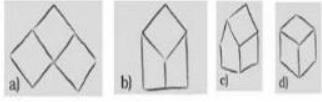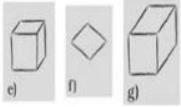Which of these drawing look correct to you?
Ans.
The drawing numbered (d) and (e) look correct.

Q.2. Which of these nets will make a cube?
(a)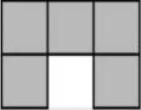(b)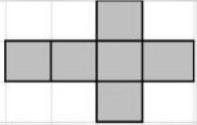(c)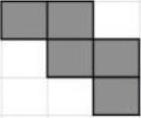Ans.
(b)

Q.3. In below shapes which figure having 12 sides and 6 faces and all of its sides are equal.
(a) Cube
(b) Triangle
(c) Square
(d) None
Ans.
Cube

Q.4. All boxes are not cubes. Here are some different types of boxes. Match the shape below with a box into which it will fold.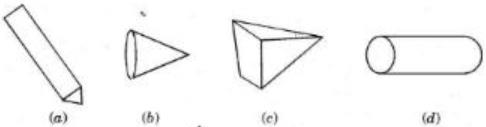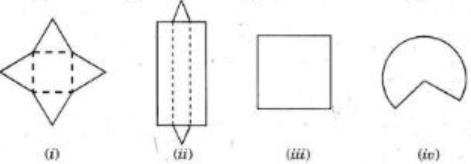Ans.
(a)-(ii), (b)-(iv), (c)-(i), (d)-(iii)

Q.5. Ramya made four shapes. Each is to be folded along the dotted lines. You have to find out which of these can be made into a box.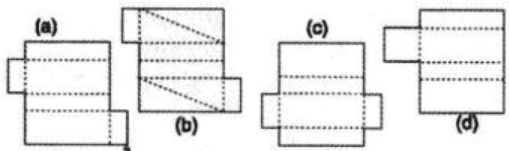Ans.
Cutouts of (a) and (c) can be folded to make boxes.

Q.6. This cut-out is folded to make a cube.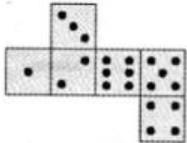Which of these are the correct deep drawings of that cube?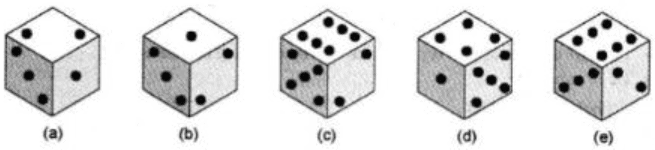Ans.
(a), (d) and (e) are the correct deep drawings.

Q.7. The cube has_____edges.
Ans.
12

Q.8. Write the formula to find the volume of the cube.
Ans.
Length x Breadth x Height

Q.9. Join the comers of the figure and write the name of the solid.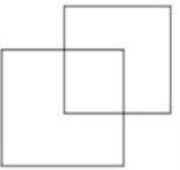Ans.
Cube

Q.10. The views below represent a three-dimensional figure that cannot be built from cubes Determine which three-dimensional figures match the views.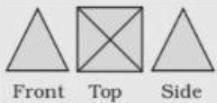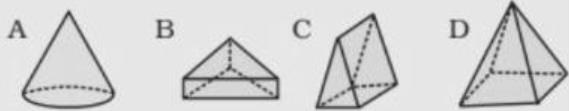Ans.
D

Q.11. Join the corners of the figure and write the name of the solid.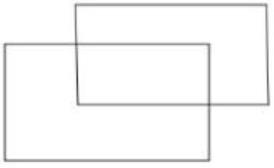Ans. Cuboid

Q.12. Count the number of cubes in the given shapes?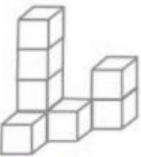Ans.
8 cubes

Q.13. Count the number of cubes in the given shapes?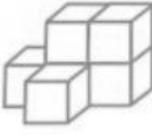Ans.
6 cubes

Q.14. Which of the nets given below will generate a cone?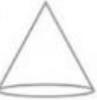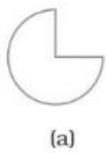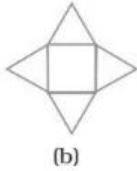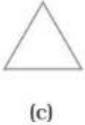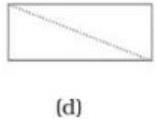Ans.
(a)

Q.15. Which of the following shapes has a vertex?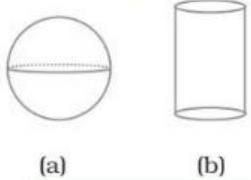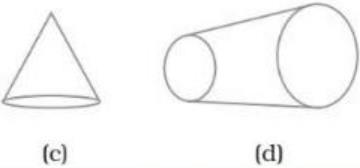Ans.
(c)

Q.16. For each of the following solids, identify the front, side and top views?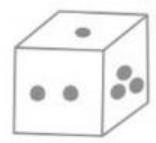(i)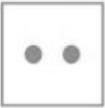(ii)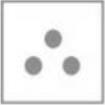(iii)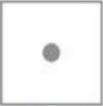Ans.
(i) - Front view (ii) - Side view, (iii) - Top view

Q.17.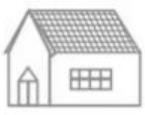(i)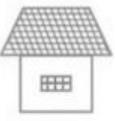(ii)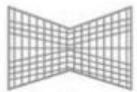(iii)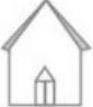Ans.
(i) - Side view, (ii) - Top view, (iii) - Front View

Q.18.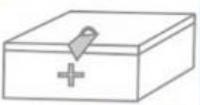(i)(ii)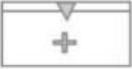(iii)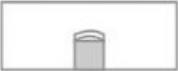Ans.
(i) - Side view, (ii) - Front view, (iii) - Top view

Q.19. Which of the following is the top view of the given shape?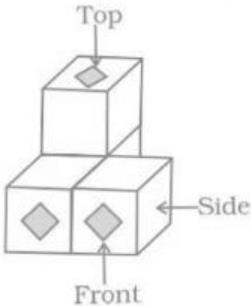(a)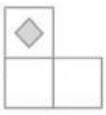(b)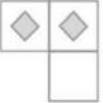(c)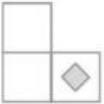(d)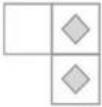Ans.
(a)

Q.20. How many vertices does a cube have?
Ans.
8

Offer running on EduRev: Apply code STAYHOME200 to get INR 200 off on our premium plan EduRev Infinity!

## Mathematics for Class 5 (V) - CBSE and NCERT Curriculum

50 videos|43 docs|39 tests

,

,

,

,

,

,

,

,

,

,

,

,

,

,

,

,

,

,

,

,

,

;